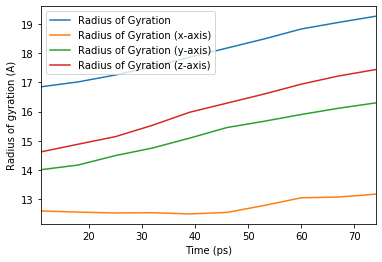# Writing your own trajectory analysis¶

We create our own analysis methods for calculating the radius of gyration of a selection of atoms.

This can be done three ways, from least to most flexible:

The building blocks and methods shown here are only suitable for analyses that involve iterating over the trajectory once.

If you implement your own analysis method, please consider contributing it to the MDAnalysis codebase!

Last executed: Feb 07, 2020 with MDAnalysis 0.20.2-dev0

Last updated: February 2020

Minimum version of MDAnalysis: 0.19.0

Packages required:

:

import MDAnalysis as mda
from MDAnalysis.tests.datafiles import PSF, DCD, DCD2
from MDAnalysis.analysis.base import (AnalysisBase,
AnalysisFromFunction,
analysis_class)
import numpy as np
import pandas as pd
import matplotlib.pyplot as plt
%matplotlib inline


Let’s start off by defining a standalone analysis function.

The radius of gyration of a structure measures how compact it is. In GROMACS, it is calculated as follows:

$R_g = \sqrt{\frac{\sum_i m_i \mathbf{r}_i^2}{\sum_i m_i}}$

where $$m_i$$ is the mass of atom $$i$$ and $$\mathbf{r}_i$$ is the position of atom $$i$$, relative to the center-of-mass of the selection.

The radius of gyration around each axis can also be determined separately. For example, the radius of gyration around the x-axis:

$R_{i, x} = \sqrt{\frac{\sum_i m_i [r_{i, y}^2 + r_{i, z}^2]}{\sum_i m_i}}$

Below, we define a function that takes an AtomGroup and calculates the radii of gyration. We could write this function to only need the AtomGroup. However, we also add in a masses argument and a total_mass keyword to avoid recomputing the mass and total mass for each frame.

:

def radgyr(atomgroup, masses, total_mass=None):
# coordinates change for each frame
coordinates = atomgroup.positions
center_of_mass = atomgroup.center_of_mass()

# get squared distance from center
ri_sq = (coordinates-center_of_mass)**2
# sum the unweighted positions
sq = np.sum(ri_sq, axis=1)
sq_x = np.sum(ri_sq[:,[1,2]], axis=1) # sum over y and z
sq_y = np.sum(ri_sq[:,[0,2]], axis=1) # sum over x and z
sq_z = np.sum(ri_sq[:,[0,1]], axis=1) # sum over x and y

# make into array
sq_rs = np.array([sq, sq_x, sq_y, sq_z])

# weight positions
rog_sq = np.sum(masses*sq_rs, axis=1)/total_mass
# square root and return
return np.sqrt(rog_sq)


The test files we will be working with here feature adenylate kinase (AdK), a phosophotransferase enzyme. ([BDPW09])

:

u = mda.Universe(PSF, DCD)
protein = u.select_atoms('protein')

u2 = mda.Universe(PSF, DCD2)


## Creating an analysis from a function¶

MDAnalysis.analysis.base.AnalysisFromFunction can create an analysis from a function that works on AtomGroups. It requires the function itself, the trajectory to operate on, and then the arguments / keyword arguments necessary for the function.

:

rog = AnalysisFromFunction(radgyr, u.trajectory,
protein, protein.masses,
total_mass=np.sum(protein.masses))
rog.run();


Running the analysis iterates over the trajectory. The output is saved in rog.results, which has the same number of rows, as frames in the trajectory.

:

rog.results.shape

:

(98, 4)

:

labels = ['all', 'x-axis', 'y-axis', 'z-axis']
for col, label in zip(rog.results.T, labels):
plt.plot(col, label=label)
plt.legend()
plt.xlabel('Frame');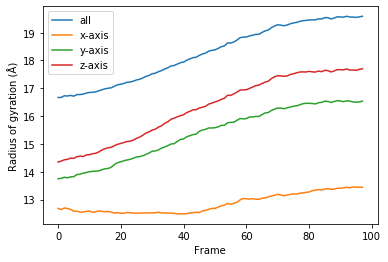You can also re-run the analysis with different frame selections.

Below, we start from the 10th frame and take every 8th frame until the 80th. Note that the slice includes the start frame, but does not include the stop frame index (much like the actual range() function).

:

rog_10 = AnalysisFromFunction(radgyr, u.trajectory,
protein, protein.masses,
total_mass=np.sum(protein.masses))

rog_10.run(start=10, stop=80, step=7)
rog_10.results.shape

:

(10, 4)

:

for col, label in zip(rog_10.results.T, labels):
plt.plot(col, label=label)
plt.legend()
plt.xlabel('Frame');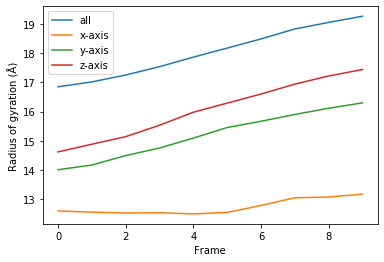## Transforming a function into a class¶

While the AnalysisFromFunction is convenient for quick analyses, you may want to turn your function into a class that can be applied to many different trajectories, much like other MDAnalysis analyses.

You can apply analysis_class to any function that you can run with AnalysisFromFunction to get a class.

:

RadiusOfGyration = analysis_class(radgyr)


To run the analysis, pass exactly the same arguments as you would for AnalysisFromFunction.

:

rog_u1 = RadiusOfGyration(u.trajectory, protein,
protein.masses,
total_mass=np.sum(protein.masses))
rog_u1.run();


As with AnalysisFromFunction, the results are in results.

:

for col, label in zip(rog_u1.results.T, labels):
plt.plot(col, label=label)
plt.legend()
plt.xlabel('Frame');You can reuse the class for other trajectories and selections.

:

ca = u2.select_atoms('name CA')

ca.masses,
total_mass=np.sum(ca.masses))
rog_u2.run();

:

for col, label in zip(rog_u2.results.T, labels):
plt.plot(col, label=label)
plt.legend()
plt.xlabel('Frame');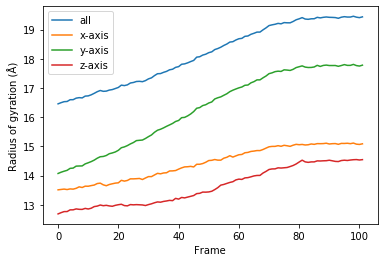Although AnalysisFromFunction and analysis_class are convenient, they can be too limited for complex algorithms. You may need to write your own class.

MDAnalysis provides the MDAnalysis.analysis.base.AnalysisBase class as a template for creating multiframe analyses. This class automatically sets up your trajectory reader for iterating, and includes an optional progress meter.

The analysis is always run by calling run(). AnalysisFromFunction actually subclasses AnalysisBase, and analysis_class returns a subclass of AnalysisFromFunction, so the behaviour of run() remains identical.

### 1. Define __init__¶

You can define a new analysis by subclassing AnalysisBase. Initialise the analysis with the __init__ method, where you must pass the trajectory that you are working with to AnalysisBase.__init__(). You can also pass in the verbose keyword. If verbose=True, the class will set up a progress meter for you.

### 2. Define your analysis in _single_frame() and other methods¶

Implement your functionality as a function over each frame of the trajectory by defining _single_frame(). This function gets called for each frame of your trajectory.

You can also define _prepare() and _conclude() to set your analysis up before looping over the trajectory, and to finalise the results that you have prepared. In order, run() calls:

• _prepare()

• _single_frame() (for each frame of the trajectory that you are iterating over)

• _conclude()

Class subclassed from AnalysisBase can make use of several properties when defining the methods above:

• self.start: frame index to start analysing from. Defined in run()

• self.stop: frame index to stop analysis. Defined in run()

• self.step: number of frames to skip in between. Defined in run()

• self.n_frames: number of frames to analyse over. This can be helpful in initialising result arrays.

• self._verbose: whether to be verbose.

• self._trajectory: the actual trajectory

• self._ts: the current timestep object

• self._frame_index: the index of the currently analysed frame. This is not the absolute index of the frame in the trajectory overall, but rather the relative index of the frame within the list of frames to be analysed. You can think of it as the number of times that self._single_frame() has already been called.

Below, we create the class RadiusOfGyration2 to run the analysis function that we have defined above, and add extra information such as the time of the corresponding frame.

:

class RadiusOfGyration2(AnalysisBase):  # subclass AnalysisBase

def __init__(self, atomgroup, verbose=True):
"""
Set up the initial analysis parameters.
"""
# must first run AnalysisBase.__init__ and pass the trajectory
trajectory = atomgroup.universe.trajectory
verbose=verbose)
# set atomgroup as a property for access in other methods
self.atomgroup = atomgroup
# we can calculate masses now because they do not depend
# on the trajectory frame.
self.masses = self.atomgroup.masses
self.total_mass = np.sum(self.masses)

def _prepare(self):
"""
Create array of zeroes as a placeholder for results.
This is run before we begin looping over the trajectory.
"""
# This must go here, instead of __init__, because
# it depends on the number of frames specified in run().
self.results = np.zeros((self.n_frames, 6))
# We put in 6 columns: 1 for the frame index,
# 1 for the time, 4 for the radii of gyration

def _single_frame(self):
"""
This function is called for every frame that we choose
in run().
"""
# call our earlier function
total_mass=self.total_mass)
# save it into self.results
self.results[self._frame_index, 2:] = rogs
# the current timestep of the trajectory is self._ts
self.results[self._frame_index, 0] = self._ts.frame
# the actual trajectory is at self._trajectory
self.results[self._frame_index, 1] = self._trajectory.time

def _conclude(self):
"""
Finish up by calculating an average and transforming our
results into a DataFrame.
"""
# by now self.result is fully populated
self.average = np.mean(self.results[:, 2:], axis=0)
columns = ['Frame', 'Time (ps)', 'Radius of Gyration',
self.df = pd.DataFrame(self.results, columns=columns)


Because RadiusOfGyration2 calculates the masses of the selected AtomGroup itself, we do not need to pass it in ourselves.

:

rog_base = RadiusOfGyration2(protein, verbose=True).run()

Step    98/98 [100.0%]


As calculated in _conclude(), the average radii of gyrations are at rog.average.

:

rog_base.average

:

array([18.26549552, 12.85342131, 15.37359575, 16.29185734])


The results are available at rog.results as an array or rog.df as a DataFrame.

:

rog_base.df

:

0 0.0 1.000000 16.669018 12.679625 13.749343 14.349043
1 1.0 2.000000 16.673217 12.640025 13.760545 14.382960
2 2.0 3.000000 16.731454 12.696454 13.801342 14.429350
3 3.0 4.000000 16.722283 12.677194 13.780732 14.444711
4 4.0 5.000000 16.743961 12.646981 13.814553 14.489046
... ... ... ... ... ... ...
93 93.0 93.999992 19.562034 13.421683 16.539112 17.653968
94 94.0 94.999992 19.560575 13.451335 16.508649 17.656678
95 95.0 95.999992 19.550571 13.445914 16.500640 17.646130
96 96.0 96.999991 19.568381 13.443243 16.507396 17.681294
97 97.0 97.999991 19.591575 13.442750 16.537926 17.704494

98 rows × 6 columns

Using this DataFrame we can easily plot our results.

:

ax = rog_base.df.plot(x='Time (ps)', y=rog_base.df.columns[2:])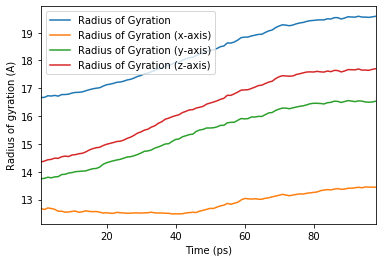We can also run the analysis over a subset of frames, the same as the output from AnalysisFromFunction and analysis_class.

:

rog_base_10 = RadiusOfGyration2(protein, verbose=True)
rog_base_10.run(start=10, stop=80, step=7);

Step    10/10 [100.0%]

:

rog_base_10.results.shape

:

(10, 6)

:

rog_base_10.df

:

0 10.0 10.999999 16.852127 12.584163 14.001589 14.614469
1 17.0 17.999998 17.019587 12.544784 14.163276 14.878262
2 24.0 24.999998 17.257429 12.514341 14.487021 15.137873
3 31.0 31.999997 17.542565 12.522147 14.747461 15.530339
4 38.0 38.999997 17.871241 12.482385 15.088865 15.977444
5 45.0 45.999996 18.182243 12.533023 15.451285 16.290153
6 52.0 52.999995 18.496493 12.771949 15.667003 16.603098
7 59.0 59.999995 18.839346 13.037335 15.900327 16.942533
8 66.0 66.999994 19.064333 13.061491 16.114195 17.222884
9 73.0 73.999993 19.276639 13.161863 16.298539 17.444213
:

ax_10 = rog_base_10.df.plot(x='Time (ps)',
y=rog_base_10.df.columns[2:])